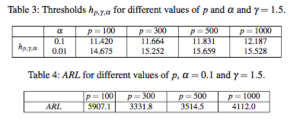A CPM-based Change Detection Test for Big Data

Thesholds and ARLs of the considered CDT have been computed through simulations since an analytical derivation of the test statistic of the CDT is hard to obtain. Thresholds have been computed as follows: for each value of p and α and γ, we simulated 10,000 experiments in which we randomly generated a p-variate normal distribution with random mean vector and covariance matrix. The threshold h is set to guarantee that the empirical probability of having a false pos- itive detection by the proposed CDT on a fixed data-sequence whose length is 2γp is equal to the confidence parameter α. Computed thresholds are ARL are here detailed.